# The Counting On Strategy for Addition

The Counting On Strategy for Addition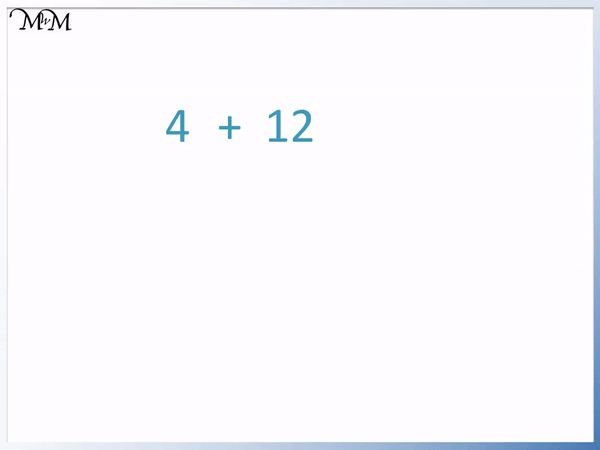• Here we are asked to add 4 and 12 using a number line.
• This means that we start at 4 and move 12 places to the right – we count on 12 more.
• It is important to make sure that we count the number of jumps until we get to 12.
• 4 + 12 = 16, however it is possible that we may make a mistake in counting 12 jumps.
• In the counting on strategy it is best to start with the largest number, which is 12.
• Instead of 4 + 12, we can look at 12 + 4, which only involves 4 jumps.
• Starting at 12 and counting on 4 jumps, we still arrive at 16.
• The order of the addition does not matter and so it is easiest to start with the largest number when using this addition strategy.
Start at the largest number in the addition on the number line.

Move right along the number line by the smallest number in the sum.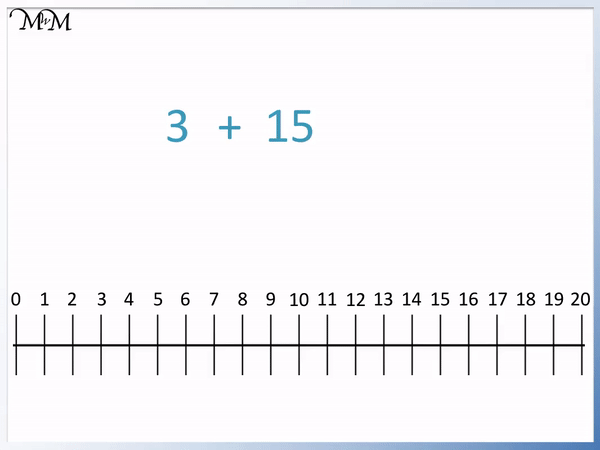• We have the sum of 3 + 15.
• In the counting on strategy we start with the largest number, so we rearrange this addition to be 15 + 3.
• We start at the largest number, which is 15 and we mark this on our number line.
• The smallest number in the addition sum is 3 and so we will make 3 jumps along the number line to the right.
• We count on 3 jumps from 15, making sure that it is the number of jumps that we count.
• We arrive at 18.
• 3 + 15 = 18.# The Counting On Strategy for Addition

## What is the Counting On Strategy?

The counting on strategy means to start with the biggest number in an addition and count on from there. For example, with 3 + 4 we start with 4 and then count on 3 more. Counting 5, 6, 7 is quicker and easier than starting at zero and counting 1, 2, 3, 4, 5, 6, 7.

The counting on strategy is a method used for improving the speed and accuracy of basic addition. It is introduced to children once they have understood the concept of numbers and their order on a number line.

The counting on strategy may appear obvious but it is not intuitive for a small child who first starts counting objects.

Here is an example of using counting on to add 4 + 3.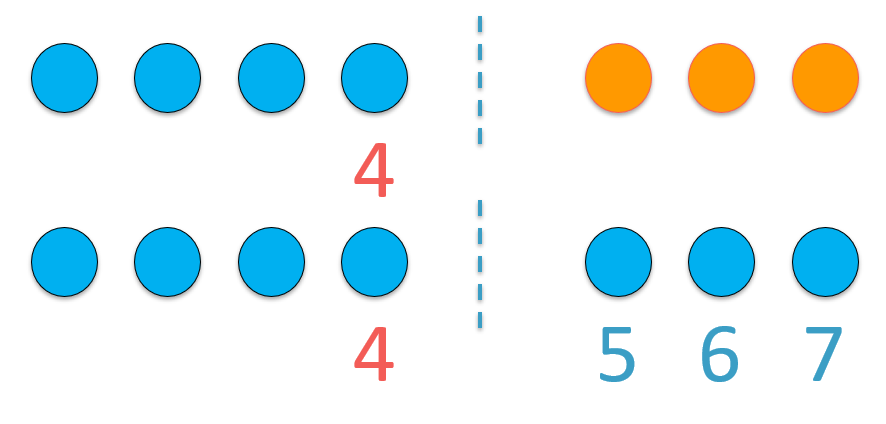We start at the largest number of 4. We then simply add 3 more from here. The next 3 numbers are 5, 6 and 7.

Therefore 4 + 3 = 7.

We will now compare this to what a child might do without learning the counting on strategy for addition.

If a child does not know how to count on, they will simply start at 0 and count the first number and then count the second number on top of this.

For example, the child would not start at 4 but instead count up from 0 to 4.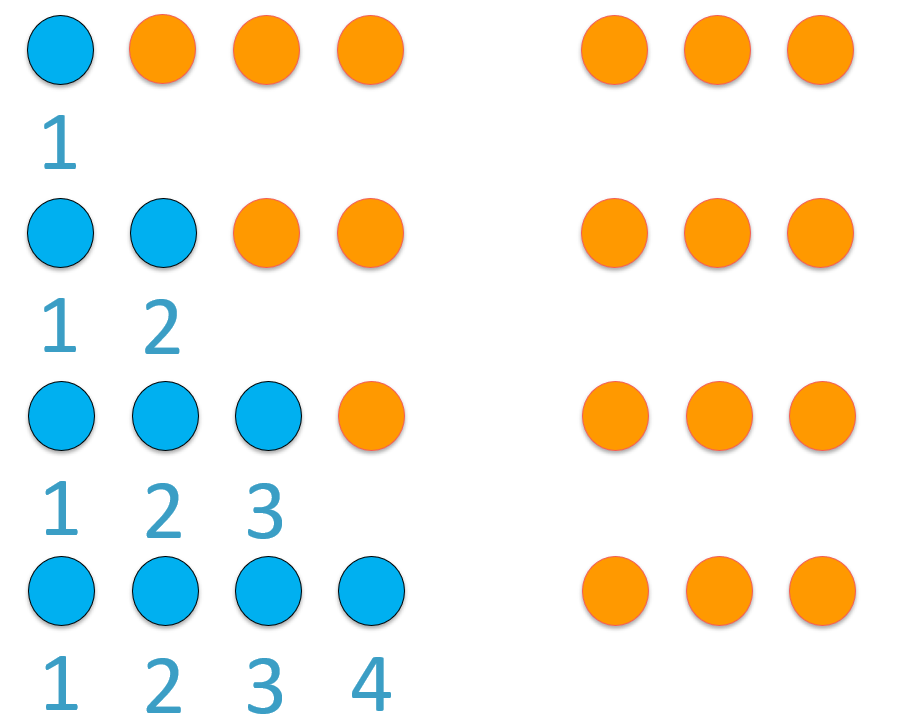Provided that we know which number comes after 4 and the sequence of numbers leading on from four, we can count on from this point.

We arrive at 7.

It is quicker to start at the larger number and count on three more.

Rather than simply counting all the way up to seven, we can start at four and count on three more.

## Counting On using a Number Line

We can use a number line to help us with the counting on addition strategy for larger numbers.

Our first example on the number line below is 4 + 12.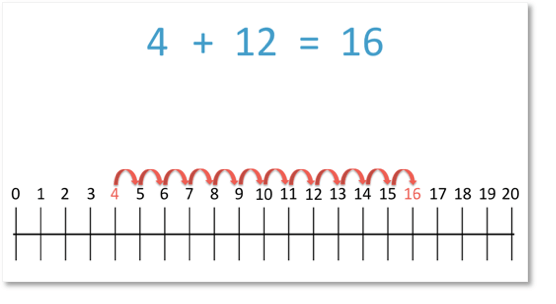To work this addition out, we could start with 4 and add on 12. However, this involves a lot of jumps on our number line and is not very efficient.

We can see that we start at 4 and make 12 jumps to arrive at 16.

We can already see that counting up to 4 and then 12 more is quite a long procedure and it is possible that we will make a mistake in our counting. It can be common for children to miscount when they are adding on larger numbers.

When using the counting on strategy, it is easier to start with the largest number and add on the smaller number.We can circle or underline the starting number to help us. In our example, we have coloured it in red.

When teaching the counting on addition method, it is important to emphasise that we are counting the number of jumps we have made to the right along the number line. We count the number of jumps after we have completed each jump and arrive at the next number.

We count 4 jumps.

This takes us to 16.

12 + 4 = 16

And so,

4 + 12 = 16

Children may commonly make mistakes with the counting procedure, particularly if they count the numbers on the line, rather than the jumps. A common misconception is that they may include the starting number as one of the 4 numbers that they have added.

It is best to reinforce that it is the number of jumps that we are counting and when teaching this, you could move your finger or a toy along the number line as you say each number, only saying each number as you arrive at it.

Here is another example of using the counting on addition strategy.

We have 4 + 14.

Remember that we do not want to be using the counting on strategy to count on with a large number. It will be more difficult to do this without making a mistake in the counting.We will instead count on from the largest number in the addition sentence.

The largest number is 14 and so we will start here.

Instead of 4 + 14, we will calculate 14 + 4.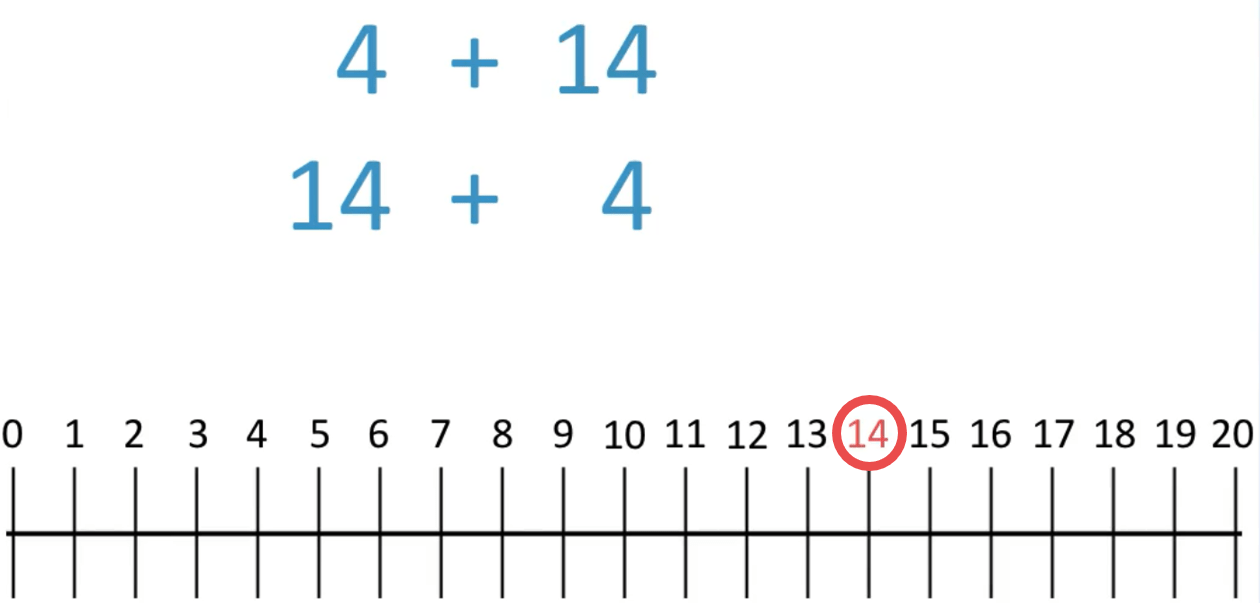Remember that we can circle, underline or highlight the starting number of 14. If working with a number line in front of you, you can put your finger or a toy at 14.

We will count on 4 more to add 4. This is counting 4 jumps to the right.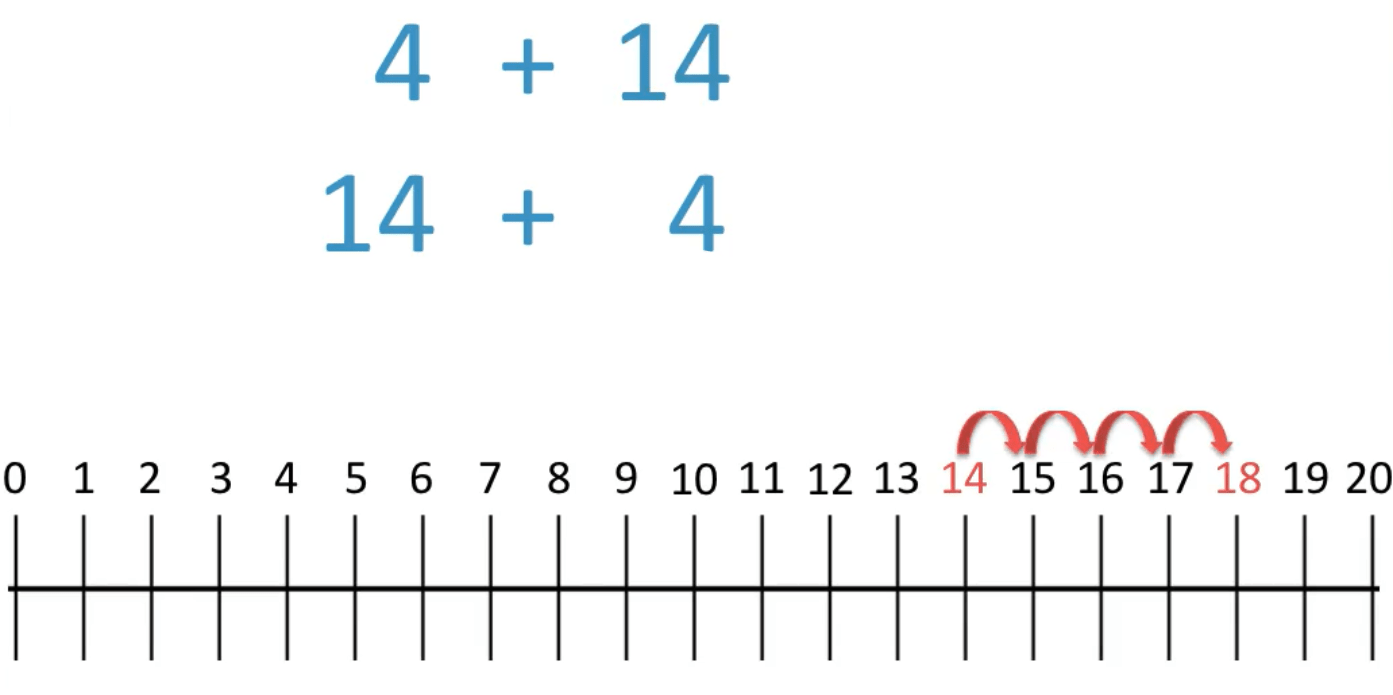We arrive at 18.

Therefore, 14 + 4 = 18

And so, 4 + 14 = 18Now try our lesson on Addition using the Compensation Strategy where we learn another addition strategy, the compensation strategy.error: Content is protected !!